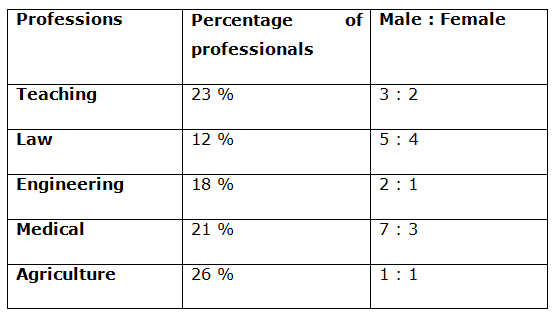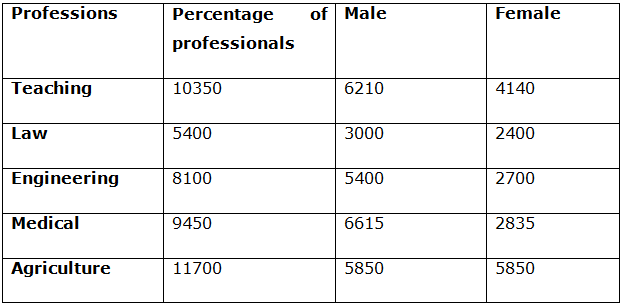# IBPS RRB Clerk/EPFO Assistant Prelims Quantitative Aptitude Questions 2019 (Day-07)

Dear Aspirants, Our IBPS Guide team is providing new series of Quantitative Aptitude Questions for IBPS RRB Clerk/EPFO Assistant Prelims 2019 so the aspirants can practice it on a daily basis. These questions are framed by our skilled experts after understanding your needs thoroughly. Aspirants can practice these new series questions daily to familiarize with the exact exam pattern and make your preparation effective.

##### EPFO Assistant Prelims Free Mock Test
[WpProQuiz 6662]

Directions (1 – 5): Find out the wrong number in the following number series.

1) 129, 130, 138, 150, 211, 236

a) 236

b) 211

c) 130

d) 150

e) 138

2) 19, 133, 798, 3980, 15960, 47880

a) 798

b) 3980

c) 15960

d) 47880

e) 133

3) 12, 26, 44, 64, 84, 107

a) 107

b) 84

c) 64

d) 44

e) 26

4) 2592, 1296, 1944, 4860, 17010, 76540

a) 1296

b) 4860

c) 17010

d) 76540

e) 1944

5) 14, 29, 88, 353, 1766, 10598

a) 88

b) 29

c) 1766

d) 353

e) 10598

Directions (6 – 10): Study the following table carefully and answer the given questions:

Following table shows the percentage of total number of professionals in different professions and the ratio of males and females among them.

Total number of professionals = 450006) Find the ratio between the total number of male professions in Teaching and Engineering to that of total number of female professions in Law and agriculture?

a) 285: 132

b) 563: 345

c) 387: 275

d) 413: 189

e) None of these

7) Find the difference between the total number of male professionals to that of total number of female professionals?

a) 8745

b) 12475

c) 14890

d) 9150

e) None of these

8) Total number of professionals in engineering and medical together is approximately total number of professionals in law and agriculture together?

a) 115 %

b) 103 %

c) 92 %

d) 84 %

e) 127 %

9) Find the average number of female professionals in all the given professions?

a) 3585

b) 4180

c) 5275

d) 2960

e) None of these

10) If the percentage of male and female teaching staffs went for a training is 30 % and 15 % respectively, then find the total number of teaching staffs doesn’t go for any training?

a) 6892

b) 8474

c) 7866

d) 5483

e) None of these

Direction (1-5) :

129 + 12 = 130

130 + 23 = 138

138 + 32 = 147

147 + 43 = 211

211 + 52 = 236

The wrong term is 150

19 * 7 = 133

133 * 6 = 798

798 * 5 = 3990

3990 * 4 = 15960

15960 * 3 = 47880

The wrong term is 3980

12 + (2 * 7) = 26

26 + (3 * 6) = 44

44 + (4 * 5) = 64

64 + (5 * 4) = 84

84 + (6 * 3) = 102

The wrong term is 107

2592 * 0.5 = 1296

1296 * 1.5 = 1944

1944 * 2.5 = 4860

4860 * 3.5 = 17010

17010 * 4.5 = 76545

The wrong term is 76540

14 * 2 + 1 = 29

29 * 3 + 1 = 88

88 * 4 + 1 = 353

353 * 5 + 1 = 1766

1766 * 6 + 1 = 10597

The wrong term is 10598

Directions (6 – 10):The total number of male professions in Teaching and Engineering

= > 6210 + 5400 = 11610

The total number of female professions in Law and agriculture

= > 2400 + 5850 = 8250

Required ratio = 11610: 8250 = 387: 275

The total number of male professionals

= > 6210 + 3000 + 5400 + 6615 + 5850

= > 27075

The total number of female professionals

= > 4140 + 2400 + 2700 + 2835 + 5850

= > 17925

Required difference = 27075 – 17925 = 9150

Total number of professionals in engineering and medical together

= > 8100 + 9450 = 17550

Total number of professionals in law and agriculture together

= > 5400 + 11700 = 17100

Required % = (17550/17100)*100 = 102.63 % = 103 %

The average number of female professionals in all the given professions

= > (4140 + 2400 + 2700 + 2835 + 5850)/5

= > 17925/5 = 3585

The total number of teaching staffs doesn’t go for any training

= > 6210*(70/100) + 4140*(85/100)

= > 4347 + 3519 = 7866